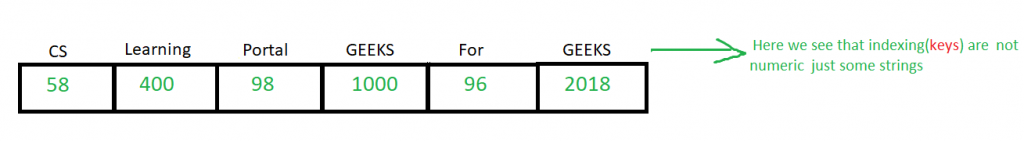Open In App

# Associative arrays in C++

Associative arrays are also called map or dictionaries. In C++. These are special kind of arrays, where indexing can be numeric or any other data type
i.e can be numeric 0, 1, 2, 3.. OR character a, b, c, d… OR string geek, computers…
These indexes are referred as keys and data stored at that position is called
value
So in associative array we have (key, value) pair.
We use STL maps to implement the concept of associative arrays in C++.Example
Now we have to print the marks of computer geeks whose names and marks are as follows

```  Name        Marks
Jessie       100
Suraj        91
Praveen      99
Bisa         78
Rithvik      84```

## CPP

 `// CPP program to demonstrate associative arrays``#include ``using` `namespace` `std;``int` `main()``{``    ``// the first data type i.e string represents``    ``// the type of key we want the second data type``    ``// i.e int represents the type of values we``    ``// want to store at that location``    ``map marks{ { "Rithvik", 78 },``            ``{ "Suraj", 91 }, { "Jessie", 100 },``            ``{ "Praveen", 99 }, { "Bisa", 84 } };` `     ``map::iterator i;``    ``cout << "The marks of all students are" << endl;``    ``for` `(i = marks.begin(); i != marks.end(); i++)``        ``cout << i->second << " ";``    `  `    ``cout << endl;` `    ``// the marks of the students based on there names.``    ``cout << "the marks of Computer geek Jessie are"``         ``<< " " << marks["Jessie"] << endl;` `    ``cout << "the marks of geeksforgeeks contributor"``          ``" Praveen are " << marks["Praveen"] << endl;``}`

Output:

```The marks of all students are
84 100 99 78 91
the marks of Computer geek Jessie are 100
the marks of geeksforgeeks ```
` Praveen are 99`